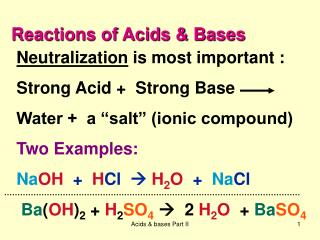DownloadDownload PresentationReactions of Acids & Bases

# Reactions of Acids & Bases

Télécharger la présentation## Reactions of Acids & Bases

- - - - - - - - - - - - - - - - - - - - - - - - - - - E N D - - - - - - - - - - - - - - - - - - - - - - - - - - -
##### Presentation Transcript

1. Reactions of Acids & Bases Neutralization is most important : Strong Acid + Strong Base Water + a “salt” (ionic compound) Two Examples: NaOH + HCl  H2O + NaCl Ba(OH)2 + H2SO4 2 H2O + BaSO4 Acids & bases Part II

2. Neutralization between weak acid & strong base is similar (weak) (strong) CH3COOH + NaOH CH3COONa + H2O CH3COONa = CH3COO– + Na+ Acids & bases Part II

3. Weak base + strong acid “salt” only NH3 + HCl NH4Cl = +NH4 + Cl– Ionic compound Acids & bases Part II

4. Buffers are mixtures of comparable amounts of a weak acid and its conjugate base (or a weak base and its conjugate acid). Examples of buffer mixtures: CH3COOH + CH3COO– 1 mole 1 mole Weak acid conjugate base Acids & bases Part II

5. NH3 + NH4+ Comparable amounts 0.5 mole 0.6 mole Weak baseconjugate acid Acids & bases Part II

6. Why the emphasis on comparable amounts of the conjugate acid-base pair? If a weak acid HA is placed in solution, most of what is present is HA. There is very little A- in solution because very little dissociation occurs for a weak acid. Acids & bases Part II

7. In the same way, when a weak base :B is put in solution, most of the material present is :B ; not much H-B+ forms because little reaction with water occurs. Acids & bases Part II

8. When a buffer is prepared we have to add extra conjugate base to a weak acid in order to make the amounts present comparable (meaning, roughly the same size). So why is this important? Acids & bases Part II

9. Scientists are interested in buffers because the pH of a buffer solution does not change very much when a small amount of another acid or base is added. Acids & bases Part II

10. The first issue is “what is the pH of the buffer?” We can answer this question by looking at the dissociation of a weak acid in water: for H-A + H2O A- + H3O+ Ka = [A-][H3O+] [HA] Acids & bases Part II

11. The expression for Ka can be rearranged by cross-multiplying to give Ka [HA] = [H3O+] [A-] This tells us that the acidity, [H3O+], depends on Ka multiplied by the ratio of [HA] and [A-]. Acids & bases Part II

12. Now if the concentrations of HA and A- in solution are comparable, this means that [HA] [A-]. Then the ratio [HA]  1 [A-] So, Ka [HA]  [H3O+] means…. [A-] Acids & bases Part II

13. Ka  [H3O+]. This means the acidity in a buffer is about the same as the dissociation constant of the weak acid. Since pH = - log [H3O+] this also means pH = - log Ka = pKa of the weak acid. Acids & bases Part II

14. Example: for acetic acid, CH3COOH, Ka = 1.75 x 10-5 (value from reference, do not memorize!) So the pH of a buffer that contains comparable amounts of CH3COOH and CH3COO- (acetate), is about 5. The actual value is pH = 4.75, so this is a good estimate. Acids & bases Part II

15. The second issue is “why does the pH of the buffer not change very much when a small amount of acid or base is added?” Let’s see… Acids & bases Part II

16. Consider a buffer mixture of acetic acid and acetate ion: CH3COOH + H2O CH3COO– + H3O+ 0.25 mole 0.25 mole Now suppose 0.02 mole HO- is added to the buffer. It reacts with and uses up 0.02 mole CH3COOH, leaving 0.23 mole. It also makes 0.02 mole CH3COO-. Acids & bases Part II

17. So now the mixture has CH3COOH + H2O CH3COO– + H3O+ 0.23 mole 0.27 mole This means for Ka [HA]  [H3O+], [A-] We have Ka(0.23/0.27)  [H3O+]. Before HO- added Ka (1/1)  [H3O+]. So: pH changes, but not very much. Acids & bases Part II

18. This is why a buffer must contain both conjugate acid-base forms. Any HO- added to a buffer reacts with the weak acid HA and makes an equal amount of conjugate base A-. The ratio [weak acid]/[conjugate base] = [HA]/[A-] changes only a little. So [H3O+] and pH do not change much as a result. Acids & bases Part II

19. Any H+ added to the buffer reacts with the conjugate base A- and makes an equal amount of HA. The ratio [HA]/[A-] does not change much and so neither does [H3O+] or pH. Acids & bases Part II